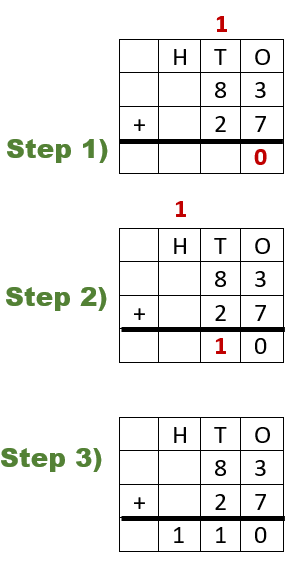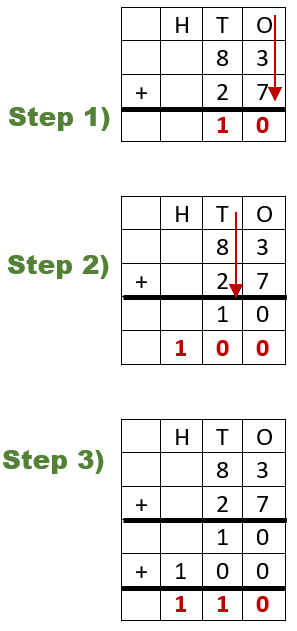# Math Worksheets Land

Math Worksheets For All Ages

# Math Worksheets Land

Math Worksheets For All Ages

Home > Math Topics > Addition >

I find that there is quite a leap when you work to help students learn to add 2-digit numbers together. They are fine with single digit and single plus double-digit addition, but you throw in two values that have two digits and to them it is totally next level. This is the sticking point skill, I promise. Once you get past this level of addition, everything pretty much comes quicker. This is because we have learned to carry and regroup while adding before this, but never at 2 possible positions (ones and tens column). This worksheet series helps students learn how to find the sum of two-digit numbers.

### Aligned Standard: 2.NBT.B.5

• Answer Keys - These are for all the unlocked materials above.

### Homework Sheets

The first two sheets offer you a helpful tip or two.

• Homework 1 - Always start at the ones column. Then move to the tens place.
• Homework 2 - If the ones column is greater than ten, carry that extra digit over to the tens place.
• Homework 3 - I like to have students place a check next to the problem, if it involves carrying to another place.

### Practice Worksheets

We arrange addends in different ways and finish off with a grid style exercise.

• Practice 1 - From right to left add the numbers and write their sum in the boxes.
• Practice 2 - We provide you with two addends.
• Practice 3 - This is a grid style worksheet.

### Math Skill Quizzes

Tens problems should give you a good indicator of what level your students are at.

• Quiz 1 - The top two problems provide you with plenty of room to work.
• Quiz 2 - This is so nuclear!
• Quiz 3 - You will need to put those two together.Adding two, two-digit numbers can be confusing for a child who just started to add numbers. Thus, for them to get used to adding double-digit (or triple-digit numbers as they advance), you can use the place-value method. Let's consider an example and we will sequentially solve them. 83 + 27 = what? Firstly, we will add 3 and 7 together to make 10. We will send the carried digit to the top of the tens value. The next step is to add the numbers to the tens place. The values are 8 + 1 + 2; which makes it 11. The total answer becomes 110.

There are a couple of different way to approach this skill with students. I have found each of them to hold a good bit of value and some work better for different students. We suggest you put all of these techniques in your bag of tricks to help you to share them with students who may be having more difficulty than most. Here are the four strategies we would suggest.

Place Break Down - I find this to be a very helpful strategy. It just requires you to break apart the values you have into nice round place values. For our example (83 + 27) we would rewrite this is tens and ones values as: ones: 3 + 7 = 10 and tens: 80 + 20 = 100. We would then add both values 10 + 100 = 110.

Numbers Line - Using a number line is not practical in most situations, but you can animate the number line a bit and fast forward the numbers a bit. You can just make a movement of ten on the number line be abbreviated. Working off of our example (83 + 27), we would need a number line with 9 intervals beyond 83 in would include 2 tens spaces, and 7 single spaces. We would only suggest this approach if students were having a ton of trouble because this is probably the last time you will sums with such low values. Number lines will no longer apply as we scale up our digits.Vertical Addition - This is simply taking what we previously did by lining up addends vertically, but we would extend the grid downward and place the sum to each column separately. In this way you avoid carrying and regrouping. If students find those difficult, it is definitely worth a try.

Solution using this strategy: Step 1) We add the ones column (3 + 7 = 10). We place that value in the next row of the grid. Step 2) We find the sum of tens place ( 80 + 20 = 100). We place that in the next row of the grid. Step 3) We simply add all of this together (10 + 100 = 110) to find our solution.

Unlock all the answers, worksheets, homework, tests and more!
Save Tons of Time! Make My Life Easier Now

## Thanks and Don't Forget To Tell Your Friends!

I would appreciate everyone letting me know if you find any errors. I'm getting a little older these days and my eyes are going. Please contact me, to let me know. I'll fix it ASAP.